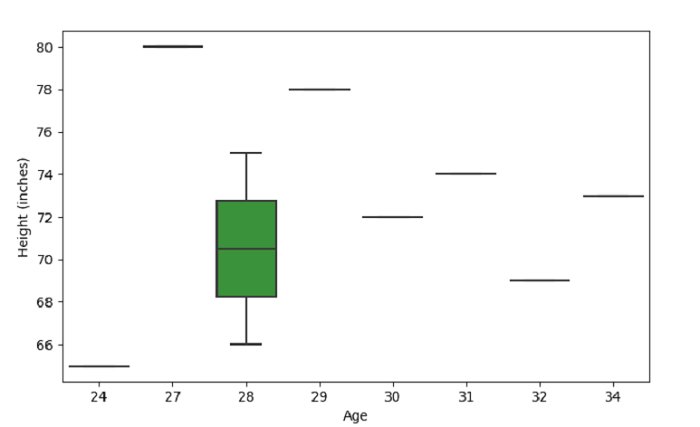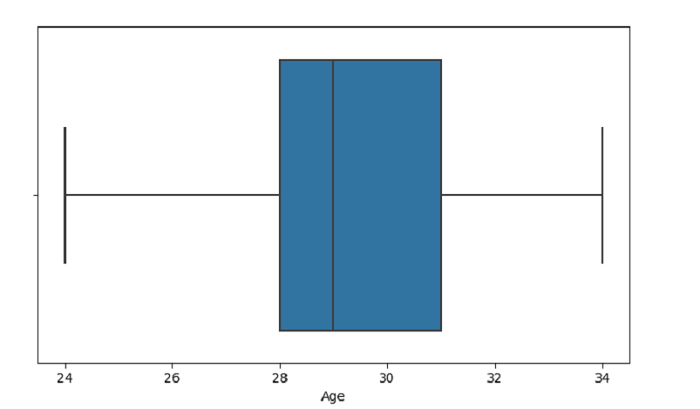# Create a Box Plot with SeaBorn â€“ Python Pandas

Box Plot in Seaborn is used to draw a box plot to show distributions with respect to categories. The seaborn.boxplot() is used for this.

Let’s say the following is our dataset in the form of a CSV file − Cricketers.csv

At first, import the required 3 libraries −

import seaborn as sb
import pandas as pd
import matplotlib.pyplot as plt

Load data from a CSV file into a Pandas DataFrame −

dataFrame = pd.read_csv("C:\Users\amit_\Desktop\Cricketers.csv")


## Example

Following is the code −

import seaborn as sb
import pandas as pd
import matplotlib.pyplot as plt

# Load data from a CSV file into a Pandas DataFrame:

# plotting box plot with Age and Heigh
sb.boxplot( dataFrame['Age'],dataFrame['Height'] )

# set label for y i.e. Height
plt.ylabel("Height (inches)")

# display
plt.show()

## Output

This will produce the following output −## Example

Let us see another example −

import seaborn as sb
import pandas as pd
import matplotlib.pyplot as plt

# Load data from a CSV file into a Pandas DataFrame:
plt.show()